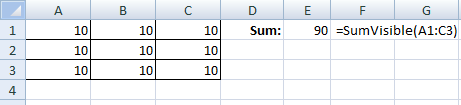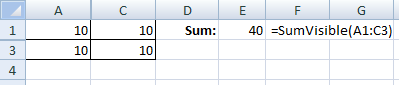# How to SUM visible cells and exclude hidden rows and columns

If you have hidden rows only, you can use SUBTOTAL function. In other cases, you can use Excel macros. Here is a VBA function that do SUM for visible cells only. It doesn’t matter if hidden are rows or columns.

`Function SumVisible(CRange As Object)`
`    Application.Volatile`
`    TotalSum = 0`
`    For Each cell In CRange`
`       If cell.Columns.Hidden = False Then`
`          If cell.Rows.Hidden = False Then`
`             TotalSum = TotalSum + cell.Value`
`          End If`
`       End If`
`    Next`
`    SumVisible = TotalSum`
`End Function`

## Example – no hidden cells

Sum range A1:C3 by the SumVisible function.## Example – hidden row and column

SumVisible A1:C3 after hiding row no.2 and B column .Note: If you hide or unhide rows or columns after you have entered the SumVisible function, you have to recalculate the worksheet. For example press F9 or do some changes in other cells.

1.2.